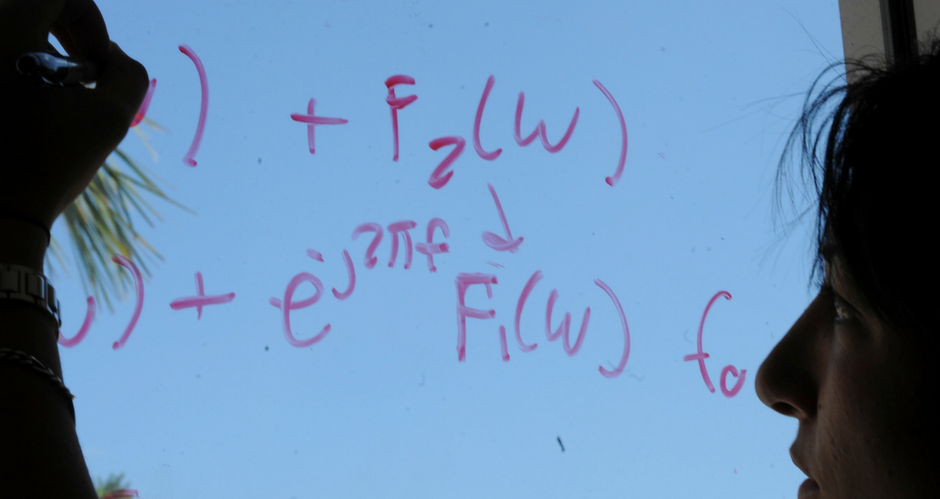## Mathematics and System Engineering Faculty Publications

### Error Bounds for Two Even Degree Tridiagonal Splines

Article

#### Publication Title

Journal of Applied Mathematics and Stochastic Analysis

#### Abstract

We study a C(¹) parabolic and a C(²) quartic spline which are determined by solution of a tridiagonal matrix and which interpolate subinterval midpoints. In contrast to the cubic C(²) spline, both of these algorithms converge to any continuous function as the length of the largest subinterval goes to zero, regardless of “mesh ratios”. For parabolic splines, this convergence property was discovered by Marsden . The quartic spline introduced here achieves this convergence by choosing the second derivative zero at the breakpoints. Many of Marsden’s bounds are substantially tightened here. We show that for functions of two or fewer coninuous derivatives the quartic spline is shown to give yet better bounds. Several of the bounds given here are optimal.

117

133

#### DOI

10.1155/S1048953390000107

1990

COinS